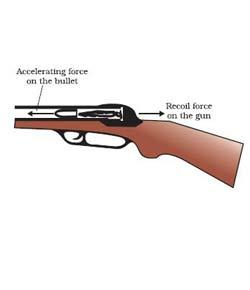# Physics Motion Online Quiz

8 Questions | Attempts: 3271
ShareSettingsPhysics is a scientific realm of study that deals with a whole host of topics such as matter, energy and force, but today we’ll be looking at a key topic in the study; motion. What can you tell us?

• 1.
A basketball is tossed up into the air. What can you say about the top of the trajectory is
• A.

Acceleration is + 9.8 m/s/s, Velocity is 0 m/s

• B.

Acceleration is 0 m/s/s, Velocity is 9.8 m/s

• C.

Acceleration is - 9.8 m/s/s, Velocity is 0 m/s

• D.

Acceleration is + 9.8 m/s, Velocity is 9.8 m/s

• E.

Acceleration is 0 m/s, Velocity is 0 m/s

• 2.
This graph is a position / time graph.Which ones are moving to the left
• A.

A

• B.

D and E

• C.

A, B, C

• D.

A, B, E

• E.

E

• 3.
A Canadian goose flew 845 km from Southern California to Oregon with an average speed of 30.5 m/s. How long, in hours, did it take the goose to make this journey?Don't forget convert the km to meters.
• A.

27.7 h

• B.

66.1 h

• C.

462 h

• D.

8.33 h

• E.

7.7 h

• 4.
A train approaches a small town with a constant velocity of +28.6 m/s. The operator applies the brake, reducing the train’s velocity to +11.4 m/s. If the average acceleration of the train during braking is –1.35 m/s2, for what elapsed time does the operator apply the brake?
• A.

8.44 s

• B.

12.7 s

• C.

3.38 s

• D.

5.92 s

• E.

10.4 s

• 5.
Starting from rest, a particle confined to move along a straight line is accelerated at a rate of 5.0 m/s2.
• A.

The particle travels 5.0 m during each second.

• B.

The particle travels 5.0 m only during the first second.

• C.

The speed of the particle increases by 5.0 m/s during each second.

• D.

The acceleration of the particle increases by 5.0 m/s2 during each second.

• E.

The final speed of the particle will be proportional to the distance that the particle covers.

• 6.
An object moving along a straight line is decelerating. Which one of the following statements concerning the object’s acceleration is necessarily true?
• A.

The value of the acceleration is positive.

• B.

The direction of the acceleration is in the same direction as the displacement.

• C.

The direction of the acceleration is in the direction opposite to that of the velocity.

• D.

The acceleration changes as the object moves along the line.

• E.

An object that is decelerating has a negative acceleration.

• 7.
The minimum takeoff speed for a certain airplane is 75 m/s. What is the acceleration for the plane must leave a 950m runway? Assume the plane starts from rest at one end of the runway.
• A.

1.5 m/s/s

• B.

3.0 m/s/s

• C.

4.5 m/s/s

• D.

6.0 m/s/s

• E.

7.5 m/s/s

• 8.
A rock is thrown vertically upward from the surface of the earth. The rock rises to some maximum height and falls back toward the surface of the earth. Which one of the following statements concerning this situation is true if air resistance is neglected?
• A.

As the ball rises, its acceleration vector points upward.

• B.

The ball is a freely falling body for the duration of its flight.

• C.

The acceleration of the ball is zero when the ball is at its highest point.

• D.

The speed of the ball is negative while the ball falls back toward the earth.

• E.

The velocity and acceleration of the ball always point in the same direction

## Related TopicsBack to top
×

Wait!
Here's an interesting quiz for you.